ICSE Class 8CISCE
Share

# Selina solutions for Class 8 Chemistry chapter 6 - Chemical Reactions

#### Selina Selina Concise Chemistry - Middle School Class 8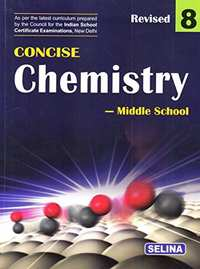## Chapter 6: Chemical Reactions

#### Selina solutions for Class 8 Chemistry Chapter 6 [Page 0]

Define a chemical reaction.

What happens during a chemical reaction ?

What do you understand by a chemical bond ?

Give one example each of which illustrates the following characteristics of a chemical reaction:

evolution of a gas

Give one example each of which illustrates the following characteristics of a chemical reaction:

change of colour

Give one example each of which illustrates the following characteristics of a chemical reaction change in state

How do the following help in bringing about a chemical change?

pressure .

How do the following help in bringing about a chemical change?

light.

How do the following help in bringing about a chemical change?

catalyst .

How do the following help in bringing about a chemical change?

heat .

Define; catalyst.

What are (i) positive catalysts and (ii) negative catalysts? Support your answer with one example for each of them.

Name three biochemical catalysts found in the human body.

What do you observe when solid lead nitrate is heated?

What do you observe when dilute sulphuric acid is added to granulated zinc?

What do you observe when a few pieces of iron are dropped in a blue solution of copper sulphate?

What do you observe when silver nitrate is added to a solution of sodium chloride?

What do you observe when ferrous sulphate solution is added to an aqueous solution of sodium hydroxide.

What do you observe when when dilute sulphuric acid is added to barium chloride solution ?

Complete and balance the following chemical equations:

N_2 + O_2   →

Complete and balance the following chemical equations:

H_2S + CI_2  →

Complete and balance the following chemical equations

Na + H_2 O   →

Complete and balance the following chemical equations

NaCI + AgNO_3  →

Complete and balance the following chemical equations:

Zn + H_2SO_4  →

(dil)

Complete and balance the following chemical equations:

Fe SO_4  + NaOH  →

(aq.)          (aq.)

Complete and balance the following chemical equations: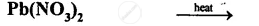Complete and balance the following chemical equations:

BaCI_2   +  H_2SO_4   →

(aq.)           (aq.)

#### Selina solutions for Class 8 Chemistry Chapter 6 [Page 0]

Fill in the blank

A reaction in which two or more substances combine to form a single substance is called a ............ reaction.

Fill in the blanK

A .............is a substance which changes the rate of a chemical reaction without undergoing a chemical change.

Fill in the blank.
The formation of gas bubbles in a liquid during a reaction is called ......................

Fill in the blank.

The reaction between an acid and a base is called ..................

Fill in the blank .

Soluble bases are called ............

Fill in the blank.

The chemical change involving iron and hydrochloric acid illustrates a ...............reaction.

Fill in the blank

In the type of reaction called ....................................., ions two compounds exchange their positive and negative radicals ions respectively.

Fill in the blank

A catalyst either ................or ............... the rate of a chemical change but itself remains ............... at the end of the reaction.

Fill in the blank

The chemical reaction between hydrogen and chlorine is a ................ reaction

Fill in the blank

When a piece of copper is added to silver nitrate solution, it turns ............in colour.

Classify the following reactions as combination .Also balance the equations.

Also balance the equations.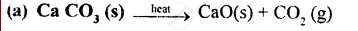(B) Zn(s) +H_2SO_4  →  ZnSO_4 (S) + H_2 (g)

(C) AgNO_3 (aq)  + NaCI (aq) → Ag CI (S) + NaNO_3

(D) NH_3 (g) + HCI (g)  → NH_4 CI (S)

(E) CuSO_4 (aq)  + H_2S (g)  → CuS (S) + H_2SO_4 (I)

(F) Zn (S) + CuSO_4 (aq)  → ZnSO_4 N  (aq) + Cu  (s)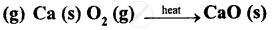(H) NaOH + HCI  → NaCI + H_2O

(I) KOH + H_2SO_4  → K_2SO_4  + H_2O

Classify the following reactions as decomposition, Also balance the equations.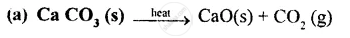(B) Zn(s) +H_2SO_4  →  ZnSO_4 (S) + H_2 (g)

(C) AgNO_3 (aq)  + NaCI (aq) → Ag CI (S) + NaNO_3

(D) NH_3 (g) + HCI (g)  → NH_4 CI (S)
(E) CuSO_4 (aq)  + H_2S (g)  → CuS (S) + H_2SO_4 (I)

(F) Zn (S) + CuSO_4 (aq)  → ZnSO_4 N  (aq) + Cu  (s)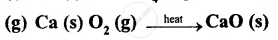(H) NaOH + HCI  → NaCI + H_2O

(I) KOH + H_2SO_4  → K_2SO_4  + H_2O

Classify the following reactions as displacement .Also balance the equations.

Also balance the equations.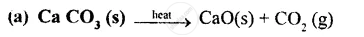(B) Zn(s) +H_2SO_4  →  ZnSO_4 (S) + H_2 (g)

(C) AgNO_3 (aq)  + NaCI (aq) → Ag CI (S) + NaNO_3

(D) NH_3 (g) + HCI (g)  → NH_4 CI (S)
(E) CuSO_4 (aq)  + H_2S (g)  → CuS (S) + H_2SO_4 (I)

(F) Zn (S) + CuSO_4 (aq)  → ZnSO_4 N  (aq) + Cu  (s)(H) NaOH + HCI  → NaCI + H_2O

(I) KOH + H_2SO_4  → K_2SO_4  + H_2O

Classify the following reactions as precipitation  .Also balance the equations.

Also balance the equations.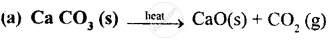(B) Zn(s) +H_2SO_4  →  ZnSO_4 (S) + H_2 (g)

(C) AgNO_3 (aq)  + NaCI (aq) → Ag CI (S) + NaNO_3

(D) NH_3 (g) + HCI (g)  → NH_4 CI (S)
(E) CuSO_4 (aq)  + H_2S (g)  → CuS (S) + H_2SO_4 (I)

(F) Zn (S) + CuSO_4 (aq)  → ZnSO_4 N  (aq) + Cu  (s)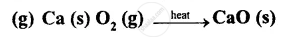(H) NaOH + HCI  → NaCI + H_2O

(I) KOH + H_2SO_4  → K_2SO_4  + H_2O

Classify the following reactions as  neutralization .Also balance the equations.

Also balance the equations.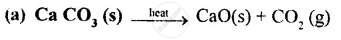(B) Zn(s) +H_2SO_4  →  ZnSO_4 (S) + H_2 (g)

(C) AgNO_3 (aq)  + NaCI (aq) → Ag CI (S) + NaNO_3

(D) NH_3 (g) + HCI (g)  → NH_4 CI (S)
(E) CuSO_4 (aq)  + H_2S (g)  → CuS (S) + H_2SO_4 (I)

(F) Zn (S) + CuSO_4 (aq)  → ZnSO_4 N  (aq) + Cu  (s)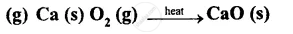(H) NaOH + HCI  → NaCI + H_2O

(I) KOH + H_2SO_4  → K_2SO_4  + H_2O

Define;

precipitation .

Define;

neutralization .

Define ;

catalyst.

Explain the following types of chemical reactions giving two examples for each of them combination reaction .

Explain the following types of chemical reactions giving two examples for each of them decomposition reaction .

Explain the following types of chemical reactions giving two examples for each of them displacement reaction.

Explain the following types of chemical reactions giving two examples for each of them  double decomposition reaction.

Write the missing reactants and products and balance the equations.

NaOH + ............... → NaCI + ...................

Write the missing reactants and products and balance the equations.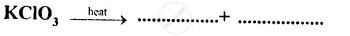Write the missing reactants and products and balance the equations.

................+ HCI → NaCI + H_2O + .........................

How will you obtain Magnesium oxide from magnesium.

Also give balanced equations for the reactions

How will you obtain  Silver chloride from silver nitrate.

Also give balanced equations for the reactions

How will you obtain  Nitrogen dioxide from lead nitrate.
Also give balanced equations for the reactions

How will you obtain Zinc chloride from zinc.

Also give balanced equations for the reactions

How will you obtain Ammonia from nitrogen.
Also give balanced equations for the reactions

What do you observe when Iron nail is kept in copper sulphate solution for sometime.

What do you observe when Phenolphthalein is added to sodium hydroxide solution.

What do you observe when Blue litmus paper is dipped in dilute hydrochloric acid.

What do you observe when Lead nitrate is heated.

What do you observe when Magnesium ribbon is burnt in oxygen.

What do you observe when Ammonia is brought in contact of hydrogen chloride.gas.

Give reason .

A person suffering from acidity is advised to take an antacid.

Give reason.

Acidic soil is treated with quick lime.

Give reason.

Wasp sting is treated with vinegar.

What is meant by the metal reactivity series ? State its importance, (any two points).

What are oxides ?

Give two examples of each of the following oxides.
Basic oxide

Give two examples of  oxides.

Acidic oxide.

Give two examples of  oxides.

Amphoteric oxide

Give two examples of oxides.

Neutral oxide

Define exothermic reactions. Give two examples of each.

Define  endothermic reactions. Give two examples of each.

State the effect of an endothermic reaction .

State the effect of  an exothermic reaction on the surroundings.

What do you observe when an acid is added to a basic solution.

What do you observe when ammonium chloride is dissolved in water.

#### Selina solutions for Class 8 Chemistry Chapter 6 [Page 0]

MULTIPLE CHOICE QUESTIONS

Which one of the following alters the rate of the chemical reaction without itself undergoing any change?

1. temperature and Pressure
2. concentration of reactants
3. presence of catalyst
4. none of these

MULTIPLE CHOICE QUESTIONS

Heat energy is evolved in these reactions

1. Thermal decomposition reactions
2. Exothermic reactions
3. Endothermic reactions
4. None of these

MULTIPLE CHOICE QUESTIONS

Thermal decomposition of a substance is brought about with the help of

1. reactants
2. water
3. wind
4. heat

MULTIPLE CHOICE QUESTIONS

Reduction reaction is the exact opposite of

1. displacement reaction
2. decomposition reaction
3. oxidation reaction
4. none of these

MULTIPLE CHOICE QUESTIONS

Which of the following is a reducing agent?

1. oxygen
2. chlorine
3. hydrogen
4. none of these

MULTIPLE CHOICE QUESTIONS

Compounds, which in their molten state allow an electric current to pass through them are called

1. electrolytes
2. non-electrolytes
3. non-conductors
4. none of these

MULTIPLE CHOICE QUESTIONS

Reduction is a chemical reaction in which there is

1. removal of hydrogen
2. removal of oxygen
4. none of these

MULTIPLE CHOICE QUESTIONS

Miscible liquids having slightly different boiling points can be separated by the

1. evaporation
2. distillation
3. fractional distillation
4. sedimentation

FILL IN THE BLANK

A .............. change is a temporary change.

FILL IN THE BLANK

In a................ reaction heat energy is absorbed.

FILL IN THE BLANK

In a double decomposition precipitation reaction both the reactants must be ............ soluble.

FILL IN THE BLANK

In a .............reaction, an acid reacts with a base to form salt and water as the only products.

FILL IN THE BLANK

Distillation is a method of obtaining pure ............. from a solution.

FILL IN THE BLANK

On reaction with sulphuric acid solution, barium chloride forms a white precipitate of ........ sulphate.

FILL IN THE BLANK

........... electrolytes dissociate completely in aqueous state and allow large amount of electricity to flow through it.

FILL IN THE BLANK

Negatively charged ions are called ..........

FILL IN THE BLANK

In a ............ change, new substances are formed.

FILL IN THE BLANK

The new substances formed during a chemical reaction are called the ..................

FILL IN THE BLANK

Positively charged ions are called ........

MATCH THE COLUMNS

 1 . H_2 + CI_2 → 2HCI a. displacement reaction 2. 2FeCI_3+ H_2  → 2FeCI_2 +2HCI b. addition of an electronegative redical 3. Fe + CuSO_4 →FeSO_4 +Cu c. removal of an electronegative redical 4. 2H_2O +4NO_2+O_2 → 4HNO_3 d.reduction by adding hydrogen 5. 3Fe +3CI_2 → 2FeCI_3 e.electrolysis of water 6. FeCI_3+ 3NaOH →Fe(OH)_3↓+NaCI F.double decomposition,precipitation reaction 7.2H_2O → 2H_2↑+O_2 e. combination or synthesis reaction

TRUE \ FALSE

A precipitation reaction takes place only in a solution.

TRUE \ FALSE

Copper can displace iron from iron sulphate.

TRUE \ FALSE

Ammonia is a good oxidizing agent.

TRUE \ FALSE

Weak electrolytes dissociate partially in their aqueous or fused state.

TRUE \ FALSE

Electrolytic refining is a method in which a thin coating of a metal is done over another metal.

TRUE \ FALSE

Evaporation of water is a physical change. True or false ?

TRUE \ FALSE

The evolution of a gas during a chemical reaction is shown by an arrow pointing downwards. True or false ?

TRUE \ FALSE

Positively charged electrode is called the anode. True or false ?

TRUE \ FALSE

Temperature at which a liquid starts boiling is known as the boiling point of that liquid. True or false ?

#### Selina solutions for Class 8 Chemistry Chapter 6 [Page 0]

What are chemical reactions?

What are biocatalysts ?

How is precipitate formation in a chemical reaction indicated?

What are homogenous reactions ?

Name the type of reaction which is governed by the position of a metal in the metal activity series ?

Define electrolysis.

Name three reducing agents ?

Is kerosene an electrolyte or a non-electrolyte ?

#### Selina solutions for Class 8 Chemistry Chapter 6 [Page 0]

What are the characteristics of a chemical reaction ? A chemical reaction is characterised by an irreversible change in which a new substance is formed.

Explain the type of reactions on the basis of the direction of reaction.

What are thermal decomposition reactions ? Explain with an example.

What are chemical combination or synthesis reactions ? Chemical combination or synthesis:

What do you mean by redox reaction ? Explain with the help of an example.

Write a short note on the information derived from a balanced chemical equation.

How is a strong electrolyte different from a weak electrolyte?

## Chapter 6: Chemical Reactions

#### Selina Selina Concise Chemistry - Middle School Class 8## Selina solutions for Class 8 Chemistry chapter 6 - Chemical Reactions

Selina solutions for Class 8 Chemistry chapter 6 (Chemical Reactions) include all questions with solution and detail explanation. This will clear students doubts about any question and improve application skills while preparing for board exams. The detailed, step-by-step solutions will help you understand the concepts better and clear your confusions, if any. Shaalaa.com has the CISCE Selina Concise Chemistry - Middle School for Class 8 solutions in a manner that help students grasp basic concepts better and faster.

Further, we at Shaalaa.com provide such solutions so that students can prepare for written exams. Selina textbook solutions can be a core help for self-study and acts as a perfect self-help guidance for students.

Concepts covered in Class 8 Chemistry chapter 6 Chemical Reactions are Types of Reactions - Combination, Types of Reactions - Decomposition, Types of Reactions - Displacement, Types of Reactions - Double Displacement, Reactivity Series - in Reactivity Series Metals Are Arranged in Order of Their Reactivity, Reactivity Series - Metal that Displaces the Metal Ion from the Solution is More Reactive., Predict the Reactivity of Metals, Endothermic and Exothermic Processes/ Reactions, Neutralization Reaction, Decomposition Reactions to Form Oxides, Classification of Oxides - Metal Oxides Are Basic, Classification of Oxides - Nonmetal Oxides Are Acidic in Nature, Classification of Oxides - Basic Oxides React with Acids, Amphoteric Oxides - Some Oxides Such as Zno, Pbo React Both with Acids and Bases, Concept for Chemical Reactions.

Using Selina Class 8 solutions Chemical Reactions exercise by students are an easy way to prepare for the exams, as they involve solutions arranged chapter-wise also page wise. The questions involved in Selina Solutions are important questions that can be asked in the final exam. Maximum students of CISCE Class 8 prefer Selina Textbook Solutions to score more in exam.

Get the free view of chapter 6 Chemical Reactions Class 8 extra questions for Chemistry and can use Shaalaa.com to keep it handy for your exam preparation

S